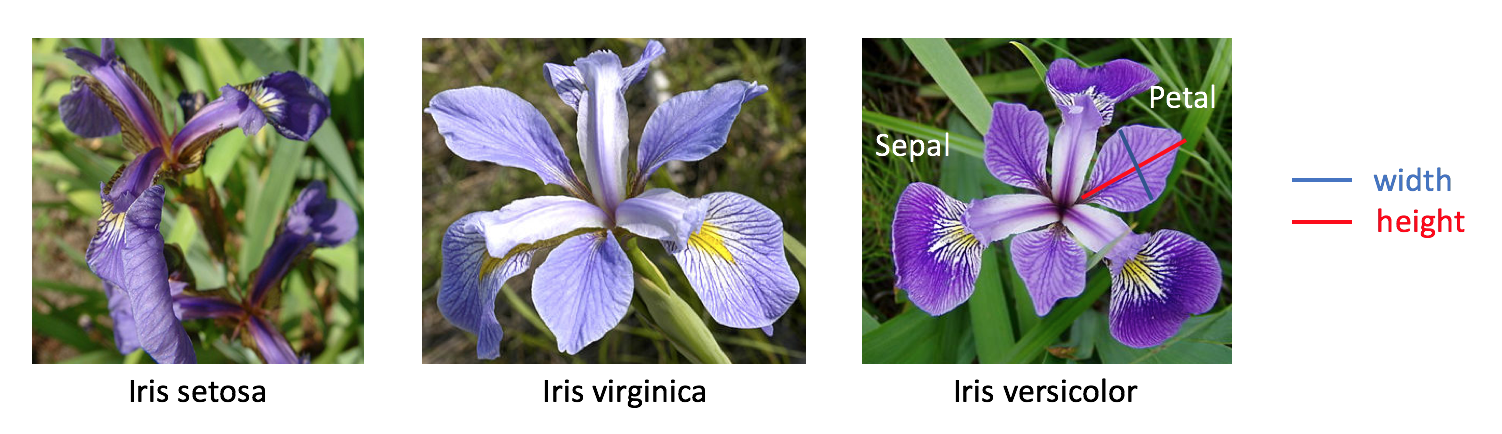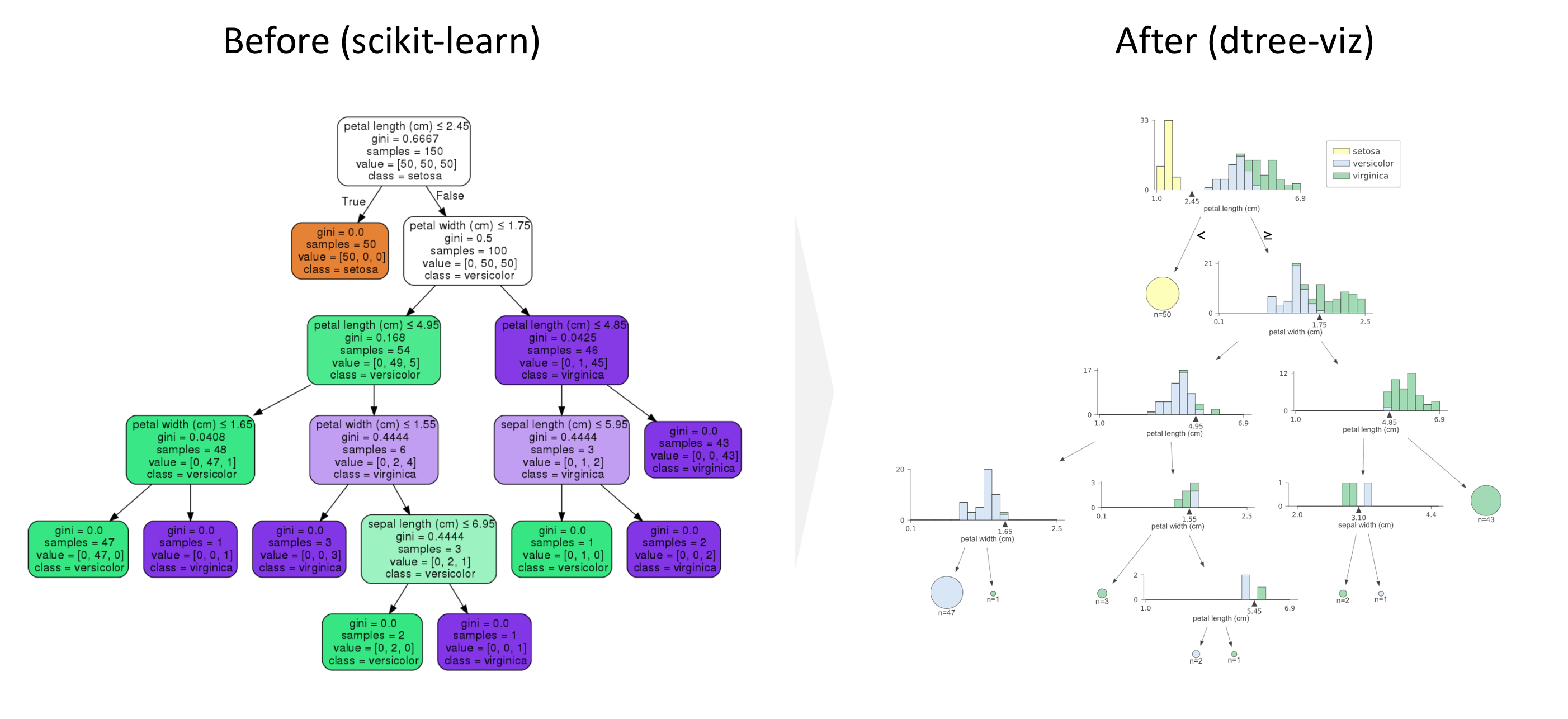## Goal¶

This post aims to introduce how to load MNIST (hand-written digit image) dataset using scikit-learn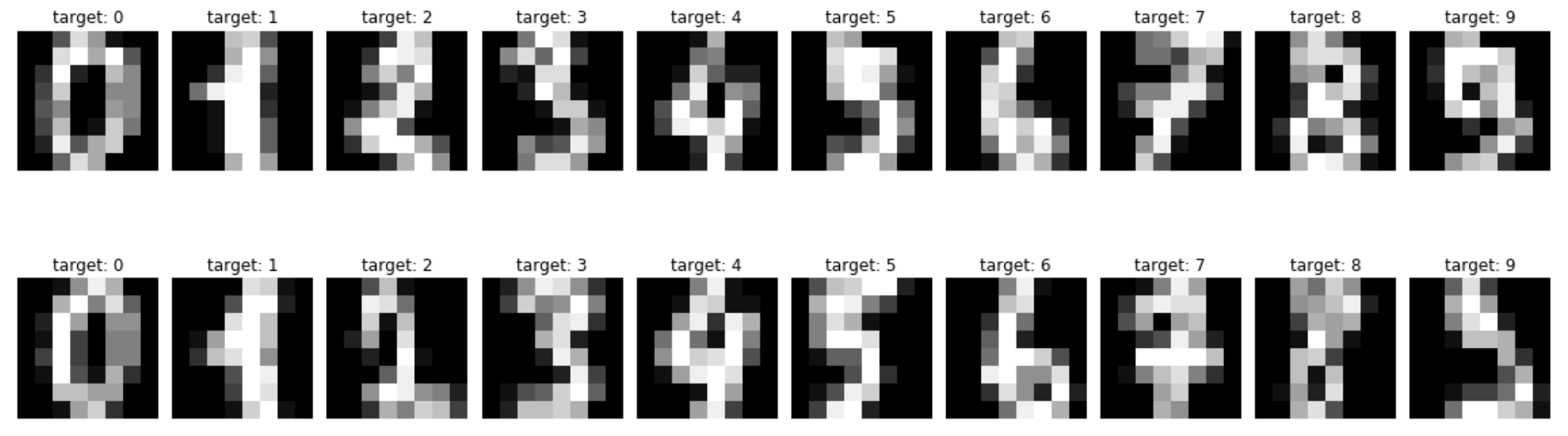Refernce

# One-Hot Encode Nominal Categorical Features

## Goal¶

This post aims to introduce how to create one-hot-encoded features for categorical variables. In this post, two ways of creating one hot encoded features: OneHotEncoder in scikit-learn and get_dummies in pandas.

Peronally, I like get_dummies in pandas since pandas takes care of columns names, type of data and therefore, it looks cleaner and simpler with less code.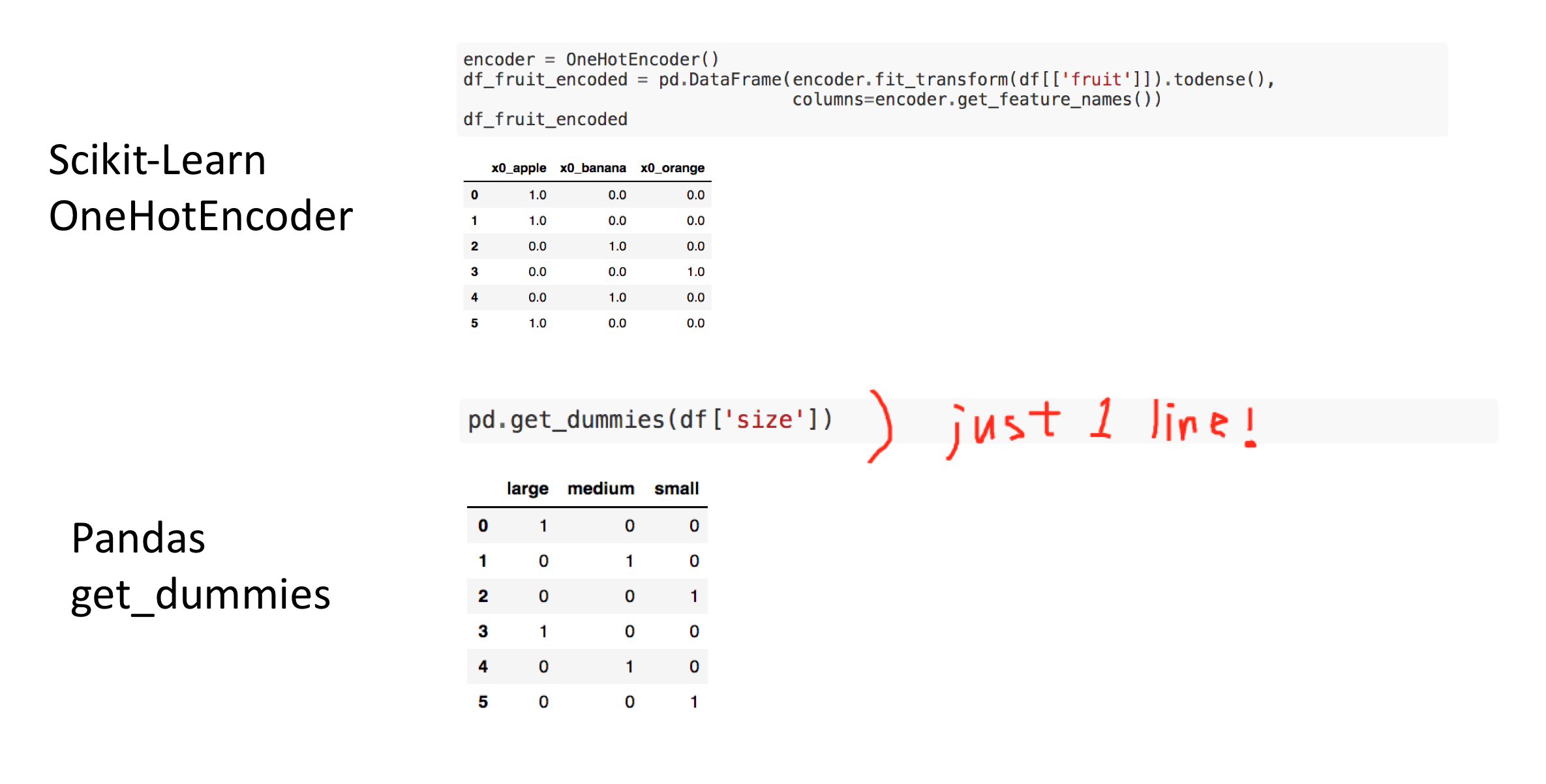Reference

# Converting A Dictionary Into A Matrix using DictVectorizer

## Goal¶

This post aims to introduce how to convert a dictionary into a matrix using DictVectorizer from scikit-learn. This is useful when you have data stored in a list of a sparse dictionary format and would like to convert it into a feature vector digestable in a scikit-learn format.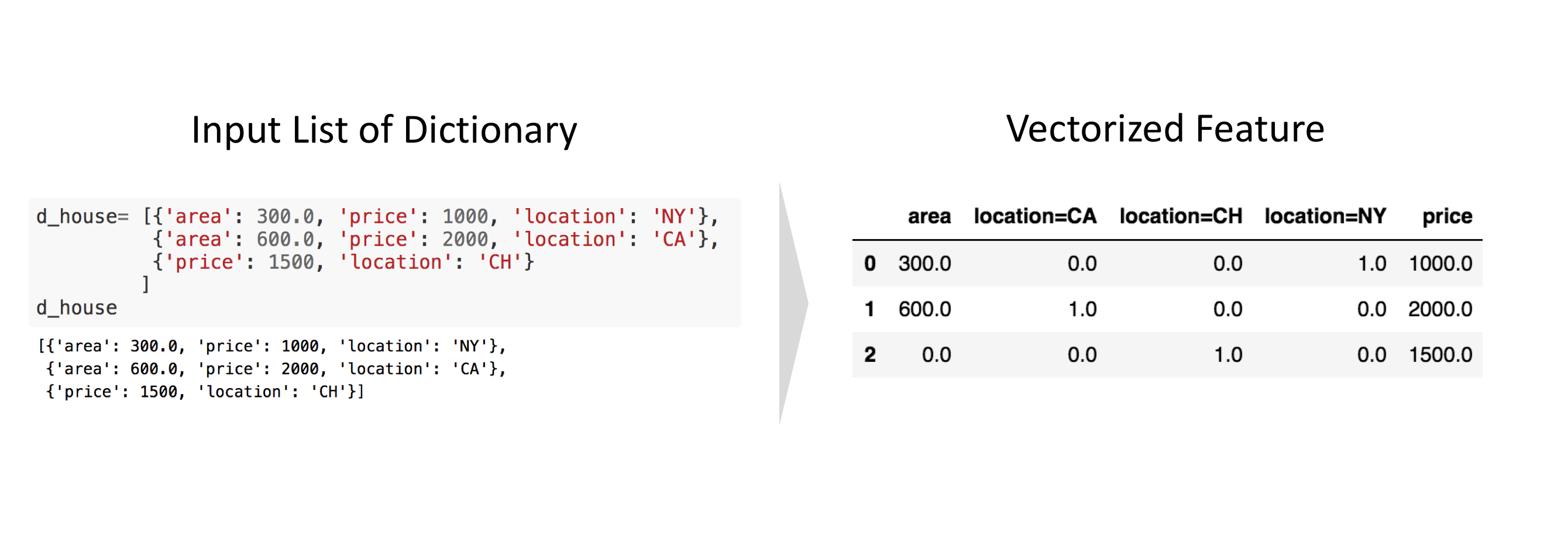Reference

## Libraries¶

In :
from sklearn.feature_extraction import DictVectorizer
import pandas as pd


## Create a list of a dictionary as an input¶

In :
d_house= [{'area': 300.0, 'price': 1000, 'location': 'NY'},
{'area': 600.0, 'price': 2000, 'location': 'CA'},
{'price': 1500, 'location': 'CH'}
]
d_house

Out:
[{'area': 300.0, 'price': 1000, 'location': 'NY'},
{'area': 600.0, 'price': 2000, 'location': 'CA'},
{'price': 1500, 'location': 'CH'}]

## Convert a list of dictionary into a feature vector¶

In :
dv = DictVectorizer()
dv.fit(d_house)

Out:
DictVectorizer(dtype=<class 'numpy.float64'>, separator='=', sort=True,
sparse=True)
In :
pd.DataFrame(dv.fit_transform(d_house).todense(), columns=dv.feature_names_)

Out:
area location=CA location=CH location=NY price
0 300.0 0.0 0.0 1.0 1000.0
1 600.0 1.0 0.0 0.0 2000.0
2 0.0 0.0 1.0 0.0 1500.0

# Logistic Regression

## Goal¶

This post aims to introduce logistic regression using dummy data.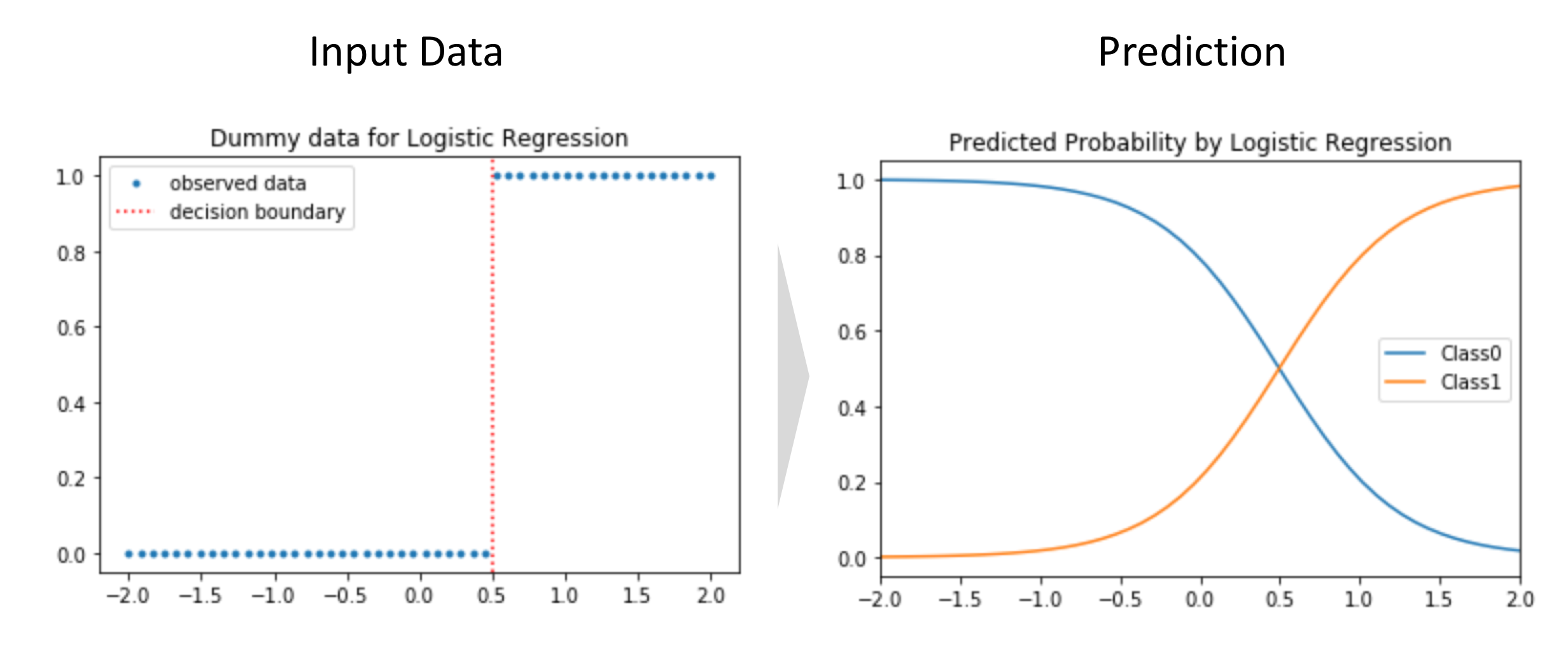Reference

# Linear Regression

## Goal¶

This post aims to introduce linear regression using dummy data.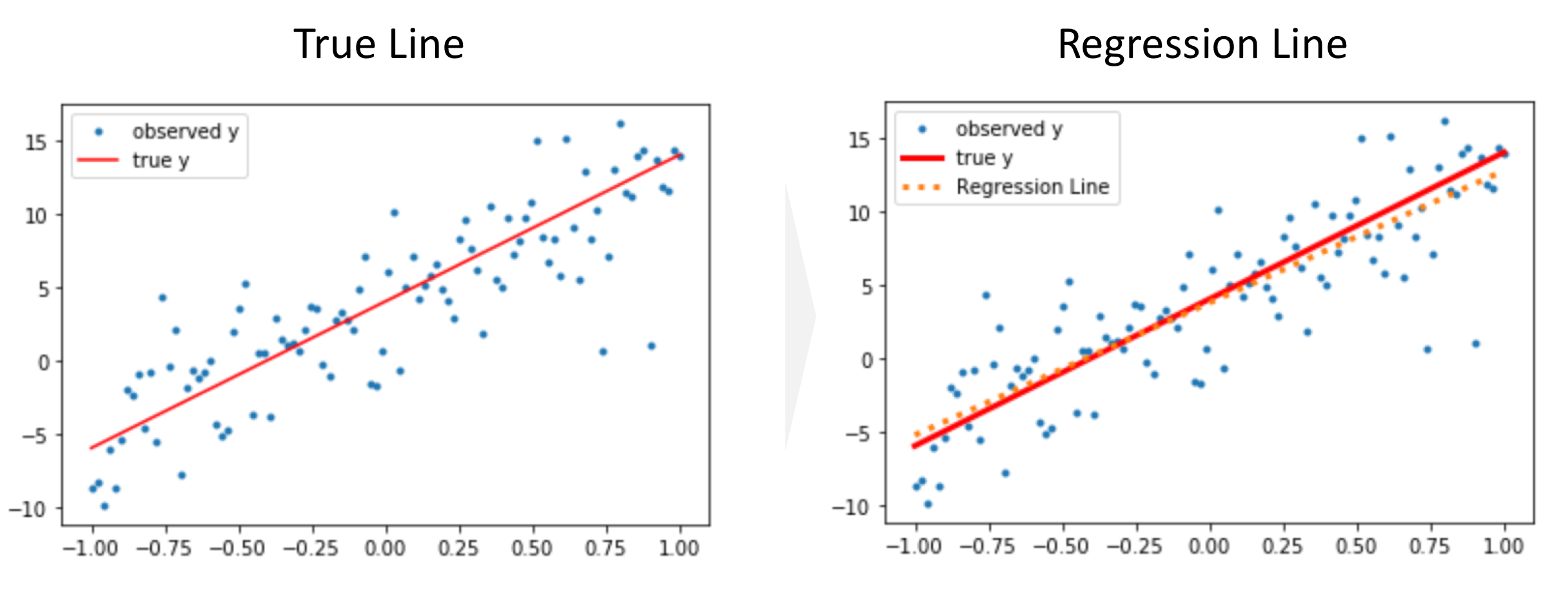Reference

## Goal¶

This post aims to introduce how to load Boston housing using scikit-learn

## Library¶

In :
from sklearn.datasets import load_boston
import pandas as pd


In :
boston = load_boston()

In :
type(boston)

Out:
sklearn.utils.Bunch
In :
boston.keys()

Out:
dict_keys(['data', 'target', 'feature_names', 'DESCR', 'filename'])

### Data¶

In :
pd.DataFrame(boston.data).head()

Out:
0 1 2 3 4 5 6 7 8 9 10 11 12
0 0.00632 18.0 2.31 0.0 0.538 6.575 65.2 4.0900 1.0 296.0 15.3 396.90 4.98
1 0.02731 0.0 7.07 0.0 0.469 6.421 78.9 4.9671 2.0 242.0 17.8 396.90 9.14
2 0.02729 0.0 7.07 0.0 0.469 7.185 61.1 4.9671 2.0 242.0 17.8 392.83 4.03
3 0.03237 0.0 2.18 0.0 0.458 6.998 45.8 6.0622 3.0 222.0 18.7 394.63 2.94
4 0.06905 0.0 2.18 0.0 0.458 7.147 54.2 6.0622 3.0 222.0 18.7 396.90 5.33

### Target¶

In :
pd.DataFrame(boston.target).head()

Out:
0
0 24.0
1 21.6
2 34.7
3 33.4
4 36.2

### Feature Name¶

In :
print(boston.feature_names)

['CRIM' 'ZN' 'INDUS' 'CHAS' 'NOX' 'RM' 'AGE' 'DIS' 'RAD' 'TAX' 'PTRATIO'
'B' 'LSTAT']


## Description¶

In :
print(boston.DESCR)

.. _boston_dataset:

Boston house prices dataset
---------------------------

**Data Set Characteristics:**

:Number of Instances: 506

:Number of Attributes: 13 numeric/categorical predictive. Median Value (attribute 14) is usually the target.

:Attribute Information (in order):
- CRIM     per capita crime rate by town
- ZN       proportion of residential land zoned for lots over 25,000 sq.ft.
- INDUS    proportion of non-retail business acres per town
- CHAS     Charles River dummy variable (= 1 if tract bounds river; 0 otherwise)
- NOX      nitric oxides concentration (parts per 10 million)
- RM       average number of rooms per dwelling
- AGE      proportion of owner-occupied units built prior to 1940
- DIS      weighted distances to five Boston employment centres
- TAX      full-value property-tax rate per $10,000 - PTRATIO pupil-teacher ratio by town - B 1000(Bk - 0.63)^2 where Bk is the proportion of blacks by town - LSTAT % lower status of the population - MEDV Median value of owner-occupied homes in$1000's

:Missing Attribute Values: None

:Creator: Harrison, D. and Rubinfeld, D.L.

This is a copy of UCI ML housing dataset.
https://archive.ics.uci.edu/ml/machine-learning-databases/housing/

This dataset was taken from the StatLib library which is maintained at Carnegie Mellon University.

The Boston house-price data of Harrison, D. and Rubinfeld, D.L. 'Hedonic
prices and the demand for clean air', J. Environ. Economics & Management,
vol.5, 81-102, 1978.   Used in Belsley, Kuh & Welsch, 'Regression diagnostics
...', Wiley, 1980.   N.B. Various transformations are used in the table on
pages 244-261 of the latter.

The Boston house-price data has been used in many machine learning papers that address regression
problems.

.. topic:: References

- Belsley, Kuh & Welsch, 'Regression diagnostics: Identifying Influential Data and Sources of Collinearity', Wiley, 1980. 244-261.
- Quinlan,R. (1993). Combining Instance-Based and Model-Based Learning. In Proceedings on the Tenth International Conference of Machine Learning, 236-243, University of Massachusetts, Amherst. Morgan Kaufmann.



# Ordinal Encoding using Scikit-learn

## Goal¶

This post aims to convert one of the categorical columns for further process using scikit-learn:

## Library¶

In :
import pandas as pd
import sklearn.preprocessing


## Create categorical data¶

In :
df = pd.DataFrame(data={'type': ['cat', 'dog', 'sheep'],
'weight': [10, 15, 50]})
df

Out:
type weight
0 cat 10
1 dog 15
2 sheep 50

## Ordinal Encoding¶

Ordinal encoding is replacing the categories into numbers.

In :
# Instanciate ordinal encoder class
oe = sklearn.preprocessing.OrdinalEncoder()

# Learn the mapping from categories to the numbers
oe.fit(df.loc[:, ['type']])

Out:
OrdinalEncoder(categories='auto', dtype=<class 'numpy.float64'>)
In :
# Apply this ordinal encoder to new data
oe.transform(pd.DataFrame(['cat'] * 3 +
['dog'] * 2 +
['sheep'] * 5))

Out:
array([[0.],
[0.],
[0.],
[1.],
[1.],
[2.],
[2.],
[2.],
[2.],
[2.]])

# How to visualize a decision tree beyond scikit-learn

## Goal¶

The goal in this post is to introduce dtreeviz to visualize a decision tree for classification more nicely than what scikit-learn can visualize. We will walk through the tutorial for decision trees in Scikit-learn using iris data set.

Note that if we use a decision tree for regression, the visualization would be different.